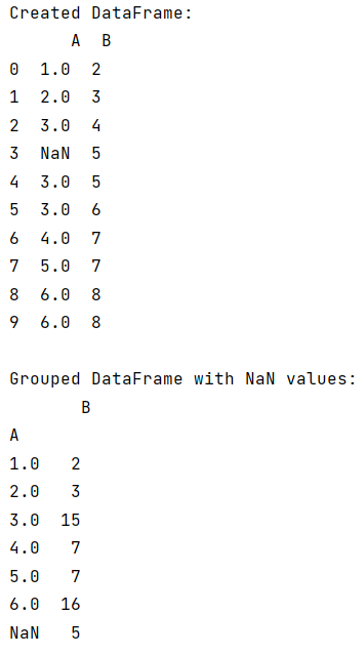# How to groupby elements of columns with NaN values?

Given a Pandas DataFrame, we have to groupby elements of columns with NaN values.
Submitted by Pranit Sharma, on June 01, 2022

While creating a DataFrame or importing a CSV file, there could be some NaN values in the cells. NaN values mean "Not-a-Number" which generally means that there are some missing values in the cell.

## Problem statement

Here, we are going to learn how to groupby column values with NaN values, as the groupby method usually excludes the NaN values hence to include NaN values, we use groupby method with some special parameters.

## Groupby elements of columns with NaN values

For this purpose, we will use pandas.DataFrame.groupby() method, this is a simple but very useful concept in pandas. By using groupby(), we can create a grouping of certain values and perform some operations on those values.

The groupby() method split the object, apply some operations, and then combines them to create a group hence a large amount of data and computations can be performed on these groups.

Syntax of pandas.DataFrame.groupby() method:

```DataFrame.groupby(
by=None,
axis=0,
level=None,
as_index=True,
sort=True,
group_keys=True,
squeeze=NoDefault.no_default,
observed=False,
dropna=True
)
```

Parameter(s):

It takes several parameters, but here we will use 'dropna = False', setting this value as False will not drop the NaN values from the column while grouping the elements.

Note

To work with pandas, we need to import pandas package first, below is the syntax:

```import pandas as pd
```

Let us understand with the help of an example,

## Python program to groupby elements of columns with NaN values

```# Importing pandas package
import pandas as pd

# Importing numpy package
import numpy as np

# Creating a Dictionary
d = {
'A':[1,2,3,np.NAN,3,3,4,5,6,6],
'B':[2,3,4,5,5,6,7,7,8,8]
}

# Creating a DataFrame
df = pd.DataFrame(d)

# Display DataFrame
print("Created DataFrame:\n",df,"\n")

# using groupby with NA values
result = df.groupby('A', dropna=False).sum()

# Display result
print("Grouped DataFrame with NaN values:\n",result)
```

## Output

The output of the above program is: